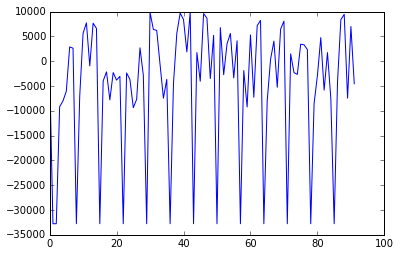I am currently doing time-series analysis on MODIS derived vegetation index data. In order to get a reliable signal from the data outliers need to be removed and the resulting gaps interpolated/filled before further filtering/smoothing of the signal. The time-series for one tile, covering 10° by 10°, spans roughly 14 years with 46 images per year. Each image weighs in at around 70-100 Mb. If you are processing, say, Africa you are looking at roughly 2.3 Terrabyte of input data. Interpolation of such massive amounts of data begs teh question - What is the fastest way to do it?

### The Question: What is the fastest way to interpolate such a massive dataset?

Each time-series needs to be interpolated in the time domain. Since every tile consists of 4800 by 4800 pixels this means the task is to interpolate 23040000 1D numpy arrays containing 644 evenly spaced data points.

My first attempts at this focussed on scipy.interp1d and the Pandas wrapper for it.

Unfortunately they turned out to be too slow to be feasible, which lead to me asking for help on StackOverflow.

### Example dataset

The example dataset reflects the input data rather well. Random Integers from -10000 to 10000 are like the NDVI dataset. There are areas where the complete z-axis is NaN (for example over water in the original data) and z-axis where only some values are NaN, just like after outlier removal along the time domain. There is high correlation along the time/z-axis. There might be some correlation in the x,y dimensions as well (Toplers Law) but this should not be employed for interpolation.

 # import necessary libraries
import numpy as np
import pandas as pd
import matplotlib.pyplot as plt
%matplotlib inline

# create example data, original is (644, 4800, 4800)
test_arr = np.random.randint(low=-10000, high=10000, size=(92, 480, 480))
test_arr[1:90:7, :, :] = -32768  # NaN fill value in original data
test_arr[2,:,:] = -32768
test_arr[:, 1:479:6, 1:479:8] = -32768

# show the example time-series at location (:,3,4)
plt.plot(test_arr[:,3,4])### Scipy.interp1d interpolation

Uitilizes scipy.signal.interp1d wrapper in pandas. This has the advantage of interpolating over a consecutive number of NaN up to a given limit with an interpolation method of choice. It is very convenient but it is rather slow.

def interpolate_nan(arr, method="linear", limit=3):
"""return array interpolated along time-axis to fill missing values"""
result = np.zeros_like(arr, dtype=np.int16)

for i in range(arr.shape):
# slice along y axis, interpolate with pandas wrapper to interp1d
line_stack = pd.DataFrame(data=arr[:,i,:], dtype=np.float32)
line_stack.replace(to_replace=-37268, value=np.NaN, inplace=True)
line_stack.interpolate(method=method, axis=0, inplace=True, limit=limit)
line_stack.replace(to_replace=np.NaN, value=-37268, inplace=True)
result[:, i, :] = line_stack.values.astype(np.int16)
return result


While this is very convenient it is way too slow for my purposes. Interpolation of my input data would require upwards of 3 weeks. I started looking for ways to speed this up. Since numpy has no fast 1D interpolation function and writing C code or learn Cython would also cost me quite some time I turned towards numba.

### 1D interpolation with numba

The idea is to loop through all 644x4800x4800 pixels and replace it with the mean of it’s neighbours in the z-axis. This kind of loop would be horribly slow in pure Python. Numba compiles this function once and thus speeds up the loop drastically.

This is done with the @jit decorator before the function. This function can also be nested into other functions as long as each one uses the decorator.

Numba is only faster than Python if it is not run in object mode. The standard behaviour is to fall back into object mode if the function can’t be compiled to low level code. The nopython=True argument supresses this behaviour and returns an exception if the code can’t be compiled. Functions have to be written in basic syntax with standard Python operations and boundary conditions have to be explicitly implemented.

This is less convenient and functional but pretty fast.

from numba import jit

@jit(nopython=True)
def interpolate_numba(arr, no_data=-32768):
"""return array interpolated along time-axis to fill missing values"""
result = np.zeros_like(arr, dtype=np.int16)

for x in range(arr.shape):
# slice along x axis
for y in range(arr.shape):
# slice along y axis
for z in range(arr.shape):
value = arr[z,y,x]
if z == 0:  # don't interpolate first value
new_value = value
elif z == len(arr[:,0,0])-1:  # don't interpolate last value
new_value = value

elif value == no_data:  # interpolate

left = arr[z-1,y,x]
right = arr[z+1,y,x]
# look for valid neighbours
if left != no_data and right != no_data:  # left and right are valid
new_value = (left + right) / 2

elif left == no_data and z == 1:  # boundary condition left
new_value = value
elif right == no_data and z == len(arr[:,0,0])-2:  # boundary condition right
new_value = value

elif left == no_data and right != no_data:  # take second neighbour to the left
more_left = arr[z-2,y,x]
if more_left == no_data:
new_value = value
else:
new_value = (more_left + right) / 2

elif left != no_data and right == no_data:  # take second neighbour to the right
more_right = arr[z+2,y,x]
if more_right == no_data:
new_value = value
else:
new_value = (more_right + left) / 2

elif left == no_data and right == no_data:  # take second neighbour on both sides
more_left = arr[z-2,y,x]
more_right = arr[z+2,y,x]
if more_left != no_data and more_right != no_data:
new_value = (more_left + more_right) / 2
else:
new_value = value
else:
new_value = value
else:
new_value = value
result[z,y,x] = int(new_value)
return result


### Comparing performance

Testing the performance of both functions on the example dataset shows that the numba function is more than 20 times faster. While it is less convenient than SciPy’s function it is easy to write a function and use numbas LLVM magic to reach speeds close to native C Code without the hassle of having to actually learn C.

%timeit interpolate_nan(test_arr)
1 loops, best of 3: 11 s per loop

%timeit interpolate_numba(test_arr)
1 loops, best of 3: 558 ms per loop